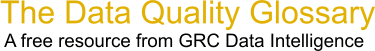Choose Index below for a list of all words and phrases defined in this glossary.

# Chi Square Test

index | Index

## Chi Square Test - definitions

Chi Square Test - The Chi Square Test is a statistical test which consists of three different types of analysis 1) Goodness of fit, 2) Test for Homogeneity, 3) Test of Independence.

The Test for Goodness of fit determines if the sample under analysis was drawn from a population that follows some specified distribution.

The Test for Homogeneity answers the proposition that several populations are homogeneous with respect to some characteristic.

The Test for independence (one of the most frequent uses of Chi Square) is for testing the null hypothesis that two criteria of classification, when applied to a population of subjects are independent. If they are not independent then there is an association between them.

Chi Square is the most popular discrete data hypothesis testing method.

[Category=Data Quality ]

Source: iSixSigma, 06 January 2011 08:02:47, http://www.isixsigma.com/index.php?option=com_glossaryThese advertisers support this free service

chi-square statistic - [statistics] A statistic used to assess how well a model fits the data. It compares categorized data with a multinomial model that predicts the relative frequency of outcomes in each category to see to what extent they agree.

[Category=Geospatial ]

Source: esri, 16 February 2012 14:46:01, http://support.esri.com/en/knowledgebase/GISDictionary/term/abbreviationData Quality Glossary.  A free resource from GRC Data Intelligence. For comments, questions or feedback: dqglossary@grcdi.nl